Ratio, proportion and percentages

This free course is available to start right now. Review the full course description and key learning outcomes and create an account and enrol if you want a free statement of participation.

Free course

1.5.1 Try some yourself

Activity 8

Which is greater, 1.2 minutes or 70 seconds?

There are at least three ways of answering this:

• (a) 70 seconds is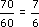= 1.1666 ... minutes, which is less than 1.2 minutes.

• (b) 1.2 minutes is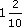=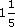minutes. 70 seconds is 1 minute 10 seconds, i.e.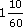=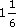minutes. Sinceis less than, 70 seconds is less than 1.2 minutes.

• (c) 1.2 minutes is 1.2 × 60 = 72 seconds, which is greater than 70 seconds.

• So 1.2 minutes is greater than 70 seconds.

Activity 9

• (a) A cheetah is the fastest land animal over short distances. It can run 400 metres in 15 seconds. What would be its speed in metres per second and kilometres per hour?

• (b) The slowest moving land animal is the three-toed sloth from tropical America. It takes 16 hours to travel a mile. What is this speed in kilometres per hour and in metres per second? (5 miles is approximately 8 km.)

• (a) Speed is the ratio of distance travelled to time taken, which in the cheetah's case is 400 metres to 15 seconds. So its speed is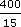27 metres per second (to 2 s.f.).

To determine this speed in kilometres per hour, both units need changing.

•   400 metres are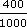kilometres

•   15 seconds are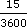hours.

• So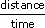in the new units is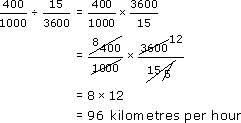• So the cheetah's speed is 96 kilometres per hour (over a distance of 400 metres).

• (b) In 16 hours the sloth moves 1 mile.

• It will take 5 × 16 = 80 hours to move 5 miles which is 8 km.

• So it will take 80 hours to move 8 km.

• In 1 hour it will move 8/80 km or 0.1 km.

• So the sloth will move at a speed of 0.1 km/h (assuming it does not fall asleep!).

• To convert this speed to metres per second,

•   0.1 km is 100 metres

•   1 hour is 3600 seconds

• So speed is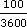0.028 metres per second (to 2 s.f.)

Activity 10

Which is longer, 11 minutes or 0.17 hours?

As a fraction of an hour, 11 minutes is 11/60 hours.

To convert this to a decimal, divide 11 by 60 to get 0.18333….

This is greater than 0.17, so 11 minutes is longer.

(Alternatively, 0.17 hours is 60 × 0.17 = 10.2 minutes, so 11 minutes is longer.)

Activity 11

A van driver averages 50 km per hour travelling on ordinary roads and 70 km per hour on motorways. Estimate:

• (i) how far the van will travel in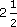hours;

• (ii) how long it will take to travel 160 km;

when travelling on (a) ordinary roads and (b) motorways.

• (a)

• (i) In 1 hour the van travels 50 km so inhours the van travels 50 ×= 125 km.

• (ii) The van travels 50 km in 1 hour so it travels 1 km in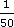hour. It therefore travels 160 km in 160 ×hours, i.e.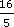hours or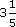hours (3 hours 12 minutes).

• (b)

• (i) 70 ×= 175 km.

• (ii)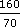2.29 hours (2 hours 17 minutes).

MU120_4M3